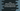# Difference between Java compareToIgnoreCase and equalsIgnoreCase## Introduction :

We can use both compareToIgnoreCase and equalsIgnoreCase to compare two strings in Java. Both methods take one string as a parameter, compare it with the caller string and return the result.

Both methods do the same thing but they differ in their return value and internal working mechanism. In this post, we will learn how these methods work and the difference between them.

### compareToIgnoreCase :

This method is defined as below :

``````public int compareToIgnoreCase(String str)
``````
• This is a public method.

• It takes one string as a parameter to compare with the caller string.

• It returns one integer value.

This method compares two strings lexicographically. The case of each character is ignored. Each character is changed to the same case by calling the below method :

Character.toLowerCase(Character.toUpperCase(character)) This method doesn’t do local sensitive comparison. That means for certain locals, it may result in an unsatisfactory ordering.

• The output will be 0 if both strings are equal.

• The output will be a positive integer if the specified string is less than the caller string.

• The output will be a negative integer if the specified string is greater than the caller string.

### equalsIgnoreCase :

This method is defined as below :

``````public boolean equalsIgnoreCase(String anotherString)
``````
• This is a public method.

• It takes one string as a parameter to compare with the caller string.

• It returns one boolean value.

It compares two strings ignoring case consideration. Two strings are called equal if they are of equal length and corresponding characters of each string are equal ignoring the case.

Two characters are considered the same ignoring case if :

• Both are same as compared by the == operator.

• The result of Character.toLowerCase(Character.toUpperCase(char)) is same for both characters.

Similar to compareToIgnoreCase method, this method doesn’t do local sensitive comparison and for certain locals, it may result in an unsatisfactory ordering.

• The output will be true if both strings are the same ignoring case.

• false otherwise.

### Difference between compareToIgnoreCase and equalsIgnoreCase :

If we compare both methods using the above definitions, the following two differences are observed :

1. compareToIgnoreCase() compare two strings lexicographically or as per dictionary ordering. But equalsIgnoreCase() checks only if both strings are equal or not.

2. The return value of compareToIgnoreCase() is an integer that represents if one string is equal, greater than or less than the other one. But equalsIgnoreCase() returns one boolean value representing if both strings are equal or not.

### Java Example Program :

``````class Example{
public static void main(String[] args){
String str1 = "Hello";
String str2 = "Hello";
String str3 = "hello";
String str4 = "mello";

//1
System.out.println(str1.compareToIgnoreCase(str2)+" "+str1.equalsIgnoreCase(str2));
//2
System.out.println(str1.compareToIgnoreCase(str3)+" "+str1.equalsIgnoreCase(str3));
//3
System.out.println(str1.compareToIgnoreCase(str4)+" "+str1.equalsIgnoreCase(str4));
}
}
``````

### Output :

``````0 true
0 true
-5 false
``````

### Explanation :

The commented numbers in the above program denote the step numbers below :

1. The first print statement printed 0 for compareToIgnoreCase and true for equalsIgnoreCase because both str1 and str2 are equal.

2. The second print statement printed 0 for compareToIgnoreCase and true for equalsIgnoreCase because both str1 and str3 are equal if we don’t consider the case of each character.

3. The third print statement printed -5 for compareToIgnoreCase and false for equalsIgnoreCase because both str1 and str4 are not equal even if we don’t consider the character case. And the difference between ‘h’ and ‘m’ ASCII value is -5.

### Conclusion :

I hope that you have found this post useful and the difference between compareToIgnoreCase and equalsIgnoreCase is clear to you. Try to run the example we have shown above and drop one comment if you have any question.